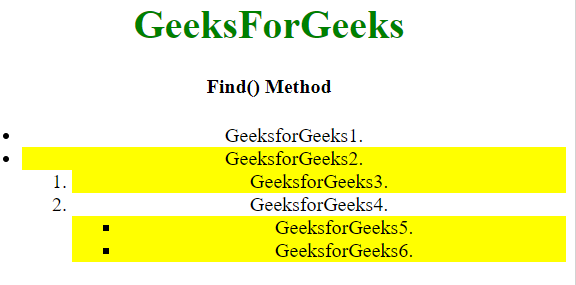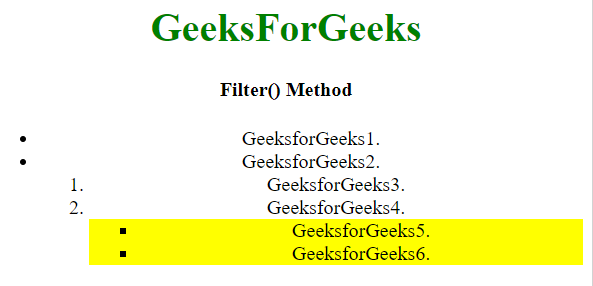Open in App
Not now

# What is the difference between find() and filter() methods in JavaScript ?

• Last Updated : 04 Apr, 2022

The find() method is used to find all the descendant elements of the selected element. It finds the element in the DOM tree by traversing through the root to leaf.

The filter() method is used to filters all the elements and returns the element that matches and the element that do not match are removed.

The only difference is the filter() method search through all the elements while find() method search through all the child elements only.

• Example 1: This example uses the find() method to search the element.

 ` ` `<``html``> ` ` `  `<``head``> ` `    ``<``script` `src``= ` `"https://ajax.googleapis.com/ajax/libs/jquery/3.4.1/jquery.min.js"``> ` `    `` ` ` `  `    ``<``script``> ` `        ``\$(document).ready(function() { ` `            ``\$("ul").find(":odd") ` `            ``.css("background-color", "yellow"); ` `        ``}); ` `    `` ` ` ` ` `  `<``body` `style``=``"text-align:center;"``> ` `     `  `    ``<``h1` `style``=``"color:green;"``>  ` `        ``GeeksForGeeks  ` `    `` ` `     `  `    ``<``h4``>Find() Method ` `     `  `    ``<``ul``> ` `        ``<``li``>GeeksforGeeks1. ` `        ``<``li``>GeeksforGeeks2. ` `        ``<``ol``> ` `            ``<``li``>GeeksforGeeks3. ` `            ``<``li``>GeeksforGeeks4. ` `            ``<``ul``> ` `                ``<``li``>GeeksforGeeks5. ` `                ``<``li``>GeeksforGeeks6. ` `            `` ` `        `` ` `    `` ` ` ` ` `  ` `

• Output:• Example 2: Changes made when we use the filter() method for searching.

 ` ` `<``html``> ` ` `  `<``head``> ` `    ``<``script` `src``= ` `"https://ajax.googleapis.com/ajax/libs/jquery/3.4.1/jquery.min.js"``> ` `    `` ` ` `  `    ``<``script``> ` `        ``\$(document).ready(function() { ` `            ``\$("ul").filter(":odd"). ` `                ``css("background-color", "yellow"); ` `        ``}); ` `    `` ` ` ` ` `  `<``body` `style``=``"text-align:center;"``> ` ` `  `    ``<``h1` `style``=``"color:green;"``>  ` `        ``GeeksForGeeks  ` `    `` ` `     `  `    ``<``h4``>Find() Method ` ` `  `    ``<``ul``> ` `        ``<``li``>GeeksforGeeks1. ` `        ``<``li``>GeeksforGeeks2. ` `        ``<``ol``> ` `            ``<``li``>GeeksforGeeks3. ` `            ``<``li``>GeeksforGeeks4. ` `            ``<``ul``> ` `                ``<``li``>GeeksforGeeks5. ` `                ``<``li``>GeeksforGeeks6. ` `            `` ` `        `` ` `    `` ` ` ` ` `  ` `

• Output:Let us see the Differences in Tabular Form -:

My Personal Notes arrow_drop_up
Related Articles